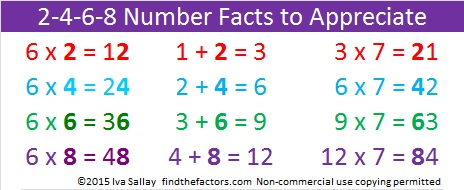# 635 Some Multiplication Facts to Know Backwards and Forwards

Something amazing happens in the multiplication table when an even number is multiplied by 6.

Something just as amazing (but backwards) happens in the multiplication table when a multiple of 3 is multiplied by 7.

Here is a graphic that may be helpful for students memorizing some of those 6 and 7 multiplication facts. It is meant to be read from left to right and contains some cool coincidences:Knowing those 6 and 7 multiplication facts will help any student know the multiplication table backwards and forwards!

While it isn’t necessary to memorize the following number facts, some of the patterns in the graphic above continue with them:

• 10 + 5 = 15, and 15 x 7 = 105
• 12 + 6 = 18, and 18 x 7 = 126
• 14 + 7 = 21, and 21 x 7 = 147
• 16 + 8 = 24, and 24 x 7 = 168
• 18 + 9 = 27, and 27 x 7 = 189

———————————————————

What makes 635 an interesting number?

635 is the sum of the nine prime numbers from 53 to 89.

It is also the sum of the thirteen prime numbers from 23 to 73.

635 can be written as the sum of 5 consecutive numbers:

125 + 126 + 127 + 128 + 129 = 635.

635 is a part of exactly 4 Pythagorean triples. Which factors of 635 are greatest common factors for the non-primitive triples?

• 635 is the hypotenuse of the Pythagorean triple 381-508-635
• 635 is the short leg in the triple 635-1524-1650
• 635 is the short leg in the triple 635-40320-40325
• 635 is the short leg in the primitive triple 635-201612-201613

———————————————————————–

• 635 is a composite number.
• Prime factorization: 635 = 5 x 127
• The exponents in the prime factorization are 1 and 1. Adding one to each and multiplying we get (1 + 1)(1 + 1) = 2 x 2 = 4. Therefore 635 has exactly 4 factors.
• Factors of 635: 1, 5, 127, 635
• Factor pairs: 635 = 1 x 635 or 5 x 127
• 635 has no square factors that allow its square root to be simplified. √635 ≈ 25.199206.## 5 thoughts on “635 Some Multiplication Facts to Know Backwards and Forwards”

1.Paula Beardell Krieg

Wow…It took me awhile to decipher what you meant by ” the product of 7 and these multiples of 3 consists of a number followed by half of itself:’Only after I got it did I see it’s accurate simplicity…it was just such a different way to think about these multiplication patterns BUT!!!! there’s more! 12 x 7 = 84, 9 x 7 = 63, 3 x 7 = 21, which all fit the pattern you are pointing out, and if you want to use a bit of creative license I suppose you could even include 0 x 7 = 00

Thanks for a puzzle of a different sort this morning.

2.Paula Beardell Krieg

OOPs! Now I see that you included the equations that I wrote down in the first table. The whole post took me longer to decipher….I started from the bottom and worked up, but not up enough. Time for more coffee 🙂

•ivasallay

I’ve edited the post and eliminated the wording you found difficult to decipher. Thank you so much for your feedback.

3.Paula Beardell Krieg

I looked at the patterns again, and OMG, since you took out that sentence I found myself being able to look AND SEE the patterns of the numbers better…I think this happened because, no longer focusing on understanding the sentence, I had more brain power left to focus on the numbers. So interesting….thank you.

•ivasallay

I’m so glad that changing it helped.

This site uses Akismet to reduce spam. Learn how your comment data is processed.